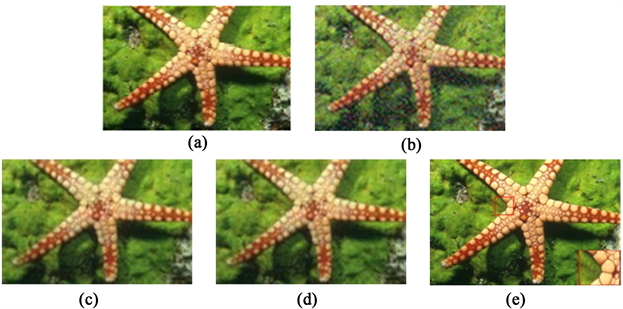﻿ 基于秩的逼近的张量鲁棒主成分分析

# 基于秩的逼近的张量鲁棒主成分分析Tensor Robust Principal Component Analysis via Non-Convex Rank Approximation

Abstract: In this paper, a new tensor rank approximation is proposed and a non-convex TRPCA model is established. An effective augmented Lagrangian multiplier optimization algorithm is used to solve this non-convex minimization problem. The experimental results show that compared with the tensor robust principal component analysis algorithm based on kernel norm, the estimated tensor obtained by this algorithm has smaller deviation and is effective in terms of accuracy and efficiency.

1. 引言

2. 预备知识

$bdiag\left(\stackrel{¯}{A}\right)=\left[\begin{array}{cccc}{\stackrel{¯}{A}}^{\left(1\right)}& & & \\ & {\stackrel{¯}{A}}^{\left(2\right)}& & \\ & & \ddots & \\ & & & {\stackrel{¯}{A}}^{\left({n}_{3}\right)}\end{array}\right]\in {C}^{{n}_{1}{n}_{3}×{n}_{2}{n}_{3}}$

$bcirc\left(\mathcal{A}\right)=\left[\begin{array}{cccc}{A}^{\left(1\right)}& {A}^{\left({n}_{3}\right)}& \cdots & {A}^{\left(2\right)}\\ {A}^{\left(2\right)}& {A}^{\left(1\right)}& \cdots & {A}^{\left(3\right)}\\ ⋮& ⋮& \ddots & ⋮\\ {A}^{\left({n}_{3}\right)}& {A}^{\left({n}_{3}-1\right)}& \cdots & {A}^{\left(1\right)}\end{array}\right]\in {C}^{{n}_{1}{n}_{3}×{n}_{2}{n}_{3}}$

$\stackrel{¯}{A}=fft\left(A,\left[\right],3\right)$

$A=U\ast S\ast {V}^{\ast }$

3. 通过秩的非凸逼近的低秩张量RPCA算法

${‖L‖}_{\gamma }=\frac{1}{{n}_{3}}\underset{i}{\sum }\underset{j}{\sum }\frac{\left(1+\gamma \right){\sigma }_{i}\left({\stackrel{¯}{L}}^{\left(j\right)}\right)}{\gamma +{\sigma }_{i}\left({\stackrel{¯}{L}}^{\left(j\right)}\right)}$ (3.1)

3.2张量RPCA的非凸优化模型

$\underset{L,S}{\mathrm{min}}{‖L‖}_{\gamma }+{‖S‖}_{{l}_{1}}\text{\hspace{0.17em}}\text{\hspace{0.17em}}\text{s}\text{.t}\text{.}\text{\hspace{0.17em}}X=L+S$ (3.2)

$L\left(L,S,Y,\mu \right)={‖L‖}_{\gamma }+\lambda {‖S‖}_{{l}_{1}}+〈Y,L+S-X〉+\frac{\mu }{2}{‖L+S-X‖}_{F}^{2}$ (3.3)

${L}_{k+1}=\underset{L}{\mathrm{arg}\mathrm{min}}{‖L‖}_{\gamma }+\frac{{\mu }^{k}}{2}{‖L-\left(X-{S}_{k}-\frac{{Y}_{k}}{{\mu }_{k}}\right)‖}_{F}^{2}$ (3.4)

${S}_{k+1}=\underset{S}{\mathrm{arg}\mathrm{min}}\lambda {‖S‖}_{{l}_{1}}+\frac{{\mu }_{k}}{2}{‖S-\left(X-{L}_{k+1}-\frac{{Y}_{k}}{{\mu }_{k}}\right)‖}_{F}^{2}$ (3.5)

${Y}_{k+1}={Y}_{k}+{\mu }_{k}\left({L}^{k+1}-X+{S}^{k+1}\right)$ (3.6)

${\mu }_{k+1}=\rho {\mu }_{k}$ (3.7)

$\underset{X}{\mathrm{min}}F\left(X\right)+\frac{\mu }{2}{‖X-A‖}_{F}^{2}$

$pro{x}_{f,\mu }\left({\sigma }_{A}\right)\underset{\sigma }{=\mathrm{arg}\mathrm{min}}f\left(\sigma \right)+\frac{\mu }{2}{‖\sigma -{\sigma }_{A}‖}_{2}^{2}$

${\sigma }_{k+1}=\mathrm{arg}\mathrm{min}〈{w}_{k},\sigma 〉+\frac{{\mu }^{t}}{2}{‖\sigma -{\sigma }_{A}‖}_{2}^{2}$

${‖\stackrel{^}{L}‖}_{\gamma }=\underset{i}{\sum }\frac{\left(1+\gamma \right){\sigma }_{i}\left(\stackrel{^}{L}\right)}{\gamma +{\sigma }_{i}\left(\stackrel{^}{L}\right)}=\underset{j}{\sum }\underset{i}{\sum }\frac{\left(1+\gamma \right){\sigma }_{i}\left({\stackrel{¯}{L}}^{\left(j\right)}\right)}{\gamma +{\sigma }_{i}\left({\stackrel{¯}{L}}^{\left( j \right)}\right)}$

$\mathrm{min}\frac{{\mu }^{k}}{2}\cdot \frac{1}{{n}_{3}}{‖\stackrel{^}{L}-\stackrel{^}{Q}‖}_{F}^{2}+\frac{1}{{n}_{3}}\cdot {‖\stackrel{^}{L}‖}_{\gamma }$ (3.8)

$\begin{array}{c}{\left(\stackrel{^}{L}\right)}^{k+1}=\underset{\stackrel{^}{L}}{\mathrm{arg}\mathrm{min}}\frac{{\mu }^{k}}{2}\cdot \frac{1}{{n}_{3}}{‖\stackrel{^}{L}-\stackrel{^}{Q}‖}_{F}^{2}+\frac{1}{{n}_{3}}\cdot {‖\stackrel{^}{L}‖}_{\gamma }\\ =\frac{1}{{n}_{3}}\underset{\stackrel{^}{L}}{\mathrm{arg}\mathrm{min}}\left\{\frac{{\mu }_{k}}{2}{‖\stackrel{^}{L}-\stackrel{^}{Q}‖}_{F}^{2}+{‖\stackrel{^}{L}‖}_{\gamma }\right\}\end{array}$ (3.9)

$pro{x}_{f,\mu }\left({\sigma }_{\stackrel{^}{Q}}\right)\underset{\sigma }{=\mathrm{arg}\mathrm{min}}f\left(\sigma \right)+\frac{\mu }{2}{‖\sigma -{\sigma }_{\stackrel{^}{Q}}‖}_{2}^{2}$ (3.10)

${\sigma }_{k+1}=\mathrm{arg}\mathrm{min}〈{w}_{k},\sigma 〉+\frac{{\mu }^{t}}{2}{‖\sigma -{\sigma }_{\stackrel{^}{Q}}‖}_{2}^{2}$ (3.11)

${\sigma }_{k+1}={\left({\sigma }_{\stackrel{^}{Q}}-\frac{{w}_{k}}{{\mu }^{t}}\right)}_{+}=\mathrm{max}\left({\sigma }_{\stackrel{^}{Q}}-\frac{{w}_{k}}{{\mu }^{t}},0\right)$ (3.12)

4. 图像数据实验Figure 1. Image recovery comparison by using different methods (a) Original image (b) Noisy images. Recovered image by (c) TRPCA (d) SNN (e) NCTRPCA, respectively

5. 结论

 Kang, Z., Peng, C. and Cheng, Q. (2015) Robust PCA via Nonconvex Rank Approximation. 2015 IEEE International Conference on Data Mining, Atlantic City, 14-17 November 2015.
https://doi.org/10.1109/ICDM.2015.15

 Boyd, S., Parikh, N., Chu, E., Peleato, B. and Eckstein, J. (2011) Distributed Optimization and Statistical Learning via the Alternating Direction Method of Multipliers. Foundations and Trends® in Machine Learning, 3, 1-122.
https://doi.org/10.1561/2200000016

 Candès, E.J., Li, X., Ma, Y. and Wright, J. (2011) Robust Principal Component Analysis? Journal of the ACM (JACM), 58, Article No. 11.
https://doi.org/10.1145/1970392.1970395

 Tao, P.D. and An, L.T.H. (1997) Convex Analysis Approach to DC Programming: Theory Algorithms and Applications. Acta Mathematica Vietnamica, 22, 289-355.

 Lu, C., Feng, J., Chen, Y., Liu, W., Lin, Z. and Yan, S. (2016) Tensor Robust Principal Component Analysis: Exact Recovery of Corrupted Low-Rank Tensors via Convex Optimization. 2016 Proceedings of the IEEE Conference on Computer Vision and Pattern Recognition, Las Vegas, 27-30 June 2016, 5249-5257.
https://doi.org/10.1109/CVPR.2016.567

 Huang, B., Mu, C., Goldfarb, D. and Wright, J. (2014) Provable Low-Rank Tensor Recovery. Optimization-Online, 4252.

Top